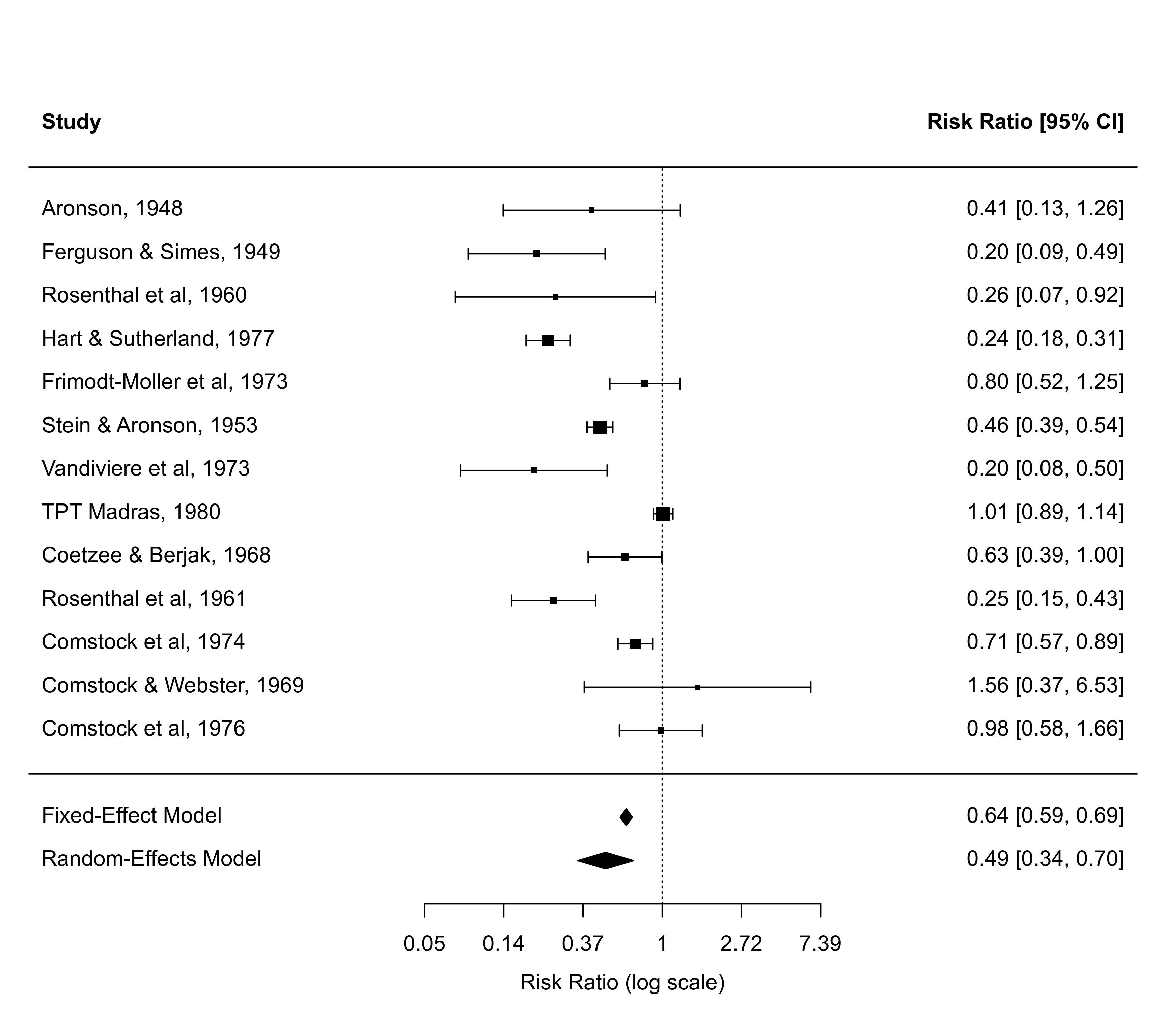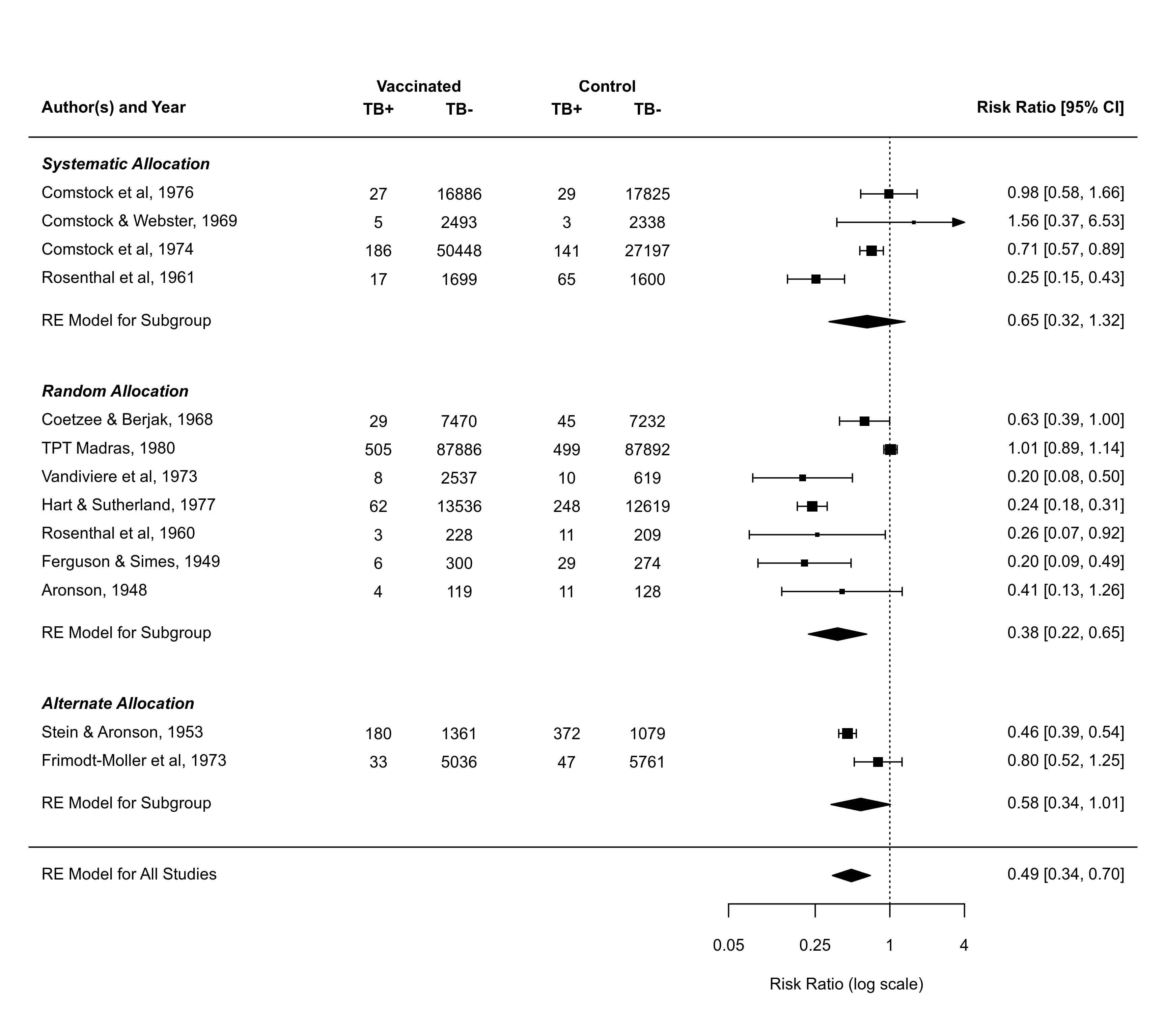Function to add a polygon to a forest plot showing the summary estimate with corresponding confidence interval based on an object of class "rma".

# S3 method for rma
addpoly(x, row=-2, level=x$level, annotate, addpred=FALSE, digits, width, mlab, transf, atransf, targs, efac, col, border, lty, fonts, cex, ...) ## Arguments x an object of class "rma". row numeric value to specify the row (or more generally, the horizontal position) for plotting the polygon (the default is -2). level numeric value between 0 and 100 to specify the confidence interval level (see here for details). The default is to take the value from the object. annotate optional logical to specify whether annotations for the summary estimate should be added to the plot. addpred logical to specify whether the bounds of the prediction interval should be added to the plot (the default is FALSE). digits optional integer to specify the number of decimal places to which the annotations should be rounded. width optional integer to manually adjust the width of the columns for the annotations. mlab optional character string giving a label for the summary estimate polygon. If unspecified, the function sets a default label. transf optional argument to specify a function to transform the summary estimate and confidence interval bound (e.g., transf=exp; see also transf). atransf optional argument to specify a function to transform the annotations (e.g., atransf=exp; see also transf). targs optional arguments needed by the function specified via transf or atransf. efac optional vertical expansion factor for the polygon. col optional character string to specify the color of the polygon. border optional character string to specify the border color of the polygon. lty optional character string to specify the line type for the prediction interval. fonts optional character string to specify the font for the label and annotations. cex optional symbol expansion factor. ... other arguments. ## Details The function can be used to add a four-sided polygon, sometimes called a summary ‘diamond’, to an existing forest plot created with the forest function. The polygon shows the summary estimate (with its confidence interval bounds) based on an equal- or a random-effects model. Using this function, summary estimates based on different types of models can be shown in the same plot. Also, summary estimates based on a subgrouping of the studies can be added to the plot this way. See ‘Examples’. If unspecified, arguments annotate, digits, width, transf, atransf, targs, efac (only if the forest plot was created with forest.rma), fonts, cex, annosym, and textpos are automatically set equal to the same values that were used when creating the forest plot. ## Author Wolfgang Viechtbauer wvb@metafor-project.org https://www.metafor-project.org ## See also forest for functions to draw forest plots to which polygons can be added. ## Examples ### meta-analysis of the log risk ratios using the Mantel-Haenszel method res <- rma.mh(measure="RR", ai=tpos, bi=tneg, ci=cpos, di=cneg, data=dat.bcg, slab=paste(author, year, sep=", ")) ### forest plot of the observed risk ratios with summary estimate forest(res, atransf=exp, xlim=c(-8,6), ylim=c(-2.5,15), header=TRUE, top=2) ### meta-analysis of the log risk ratios using a random-effects model res <- rma(measure="RR", ai=tpos, bi=tneg, ci=cpos, di=cneg, data=dat.bcg) ### add summary estimate from the random-effects model to the forest plot addpoly(res)### forest plot with subgrouping of studies and summaries per subgroup res <- rma(measure="RR", ai=tpos, bi=tneg, ci=cpos, di=cneg, data=dat.bcg, slab=paste(author, year, sep=", ")) tmp <- forest(res, xlim=c(-16, 4.6), at=log(c(0.05, 0.25, 1, 4)), atransf=exp, ilab=cbind(tpos, tneg, cpos, cneg), ilab.xpos=c(-9.5,-8,-6,-4.5), cex=0.75, ylim=c(-1, 27), order=alloc, rows=c(3:4,9:15,20:23), mlab="RE Model for All Studies", header="Author(s) and Year") op <- par(cex=0.75, font=2) text(c(-9.5,-8,-6,-4.5), tmp$ylim-1, c("TB+", "TB-", "TB+", "TB-"))
text(c(-8.75,-5.25),     tmp\$ylim,   c("Vaccinated", "Control"))
par(font=4)
text(-16, c(24,16,5), c("Systematic Allocation", "Random Allocation",
"Alternate Allocation"), pos=4)
par(op)
res <- rma(measure="RR", ai=tpos, bi=tneg, ci=cpos, di=cneg, data=dat.bcg,
subset=(alloc=="systematic"))
addpoly(res, row=18.5, mlab="RE Model for Subgroup")
res <- rma(measure="RR", ai=tpos, bi=tneg, ci=cpos, di=cneg, data=dat.bcg,
subset=(alloc=="random"))
addpoly(res, row=7.5, mlab="RE Model for Subgroup")
res <- rma(measure="RR", ai=tpos, bi=tneg, ci=cpos, di=cneg, data=dat.bcg,
subset=(alloc=="alternate"))
addpoly(res, row=1.5, mlab="RE Model for Subgroup")